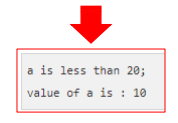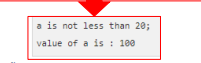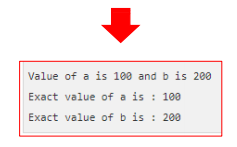# # Controlo de Fluxo

## # Instruções IF-ELSE e SWITCH

As instruções IF-ELSE e SWITCH permitem avaliar condições e desse modo condicionar o fluxo do programa.### # Instrução IF

An if statement consists of a boolean expression followed by one or more statements.``````if(boolean_expression) {
/* statement(s) will execute if the boolean expression is true*/
}
``````
``````#include <stdio.h>
int main () {

/* local variable definition */
int a = 10;

/* check the boolean condition using if statement */
if( a < 20 ) {
/* if condition is true then print the following */
printf("a is less than 20\n");
}

printf("value of a is : %d\n", a);

return 0;
}
``````### # Instrução IF-ELSE

An if statement can be followed by an optional else statement, which executes when the Boolean expression is false.``````if(boolean_expression) {
/* statement(s) will execute if the boolean expression is true*/
} else {
/* statement(s) will execute if the boolean expression is false*/
}
``````
``````#include <stdio.h>
int main () {

/* local variable definition */
int a = 100;

/* check the boolean condition using if statement */
if( a < 20 ) {
/* if condition is true then print the following */
printf("a is less than 20\n");
} else {
/* if condition is false then print the following */
printf("a is not less than 20\n");
}

printf("value of a is : %d\n", a);

return 0;
}
``````You can use one if or else if statement inside another if or else if statement(s).

``````if(boolean_expression 1) {
/* Executes when the boolean expression 1 is true */
} else if( boolean_expression 2) {
/* Executes when the boolean expression 2 is true */
} else if( boolean_expression 3) {
/* Executes when the boolean expression 3 is true */
} else {
/* executes whem none of the conditions above is true */
}

``````
``````#include <stdio.h>
int main () {

/* local variable definition */
int a = 100;

/* check the boolean condition using if statement */
if( a == 10 ) {
/* if condition is true then print the following */
printf("Value of a us 10\n");
} else if( a == 20) {
/* if else if condition is true */
printf("Value of a is 20\n");
} else if (a == 30) {
/* if else if condition is true */
printf("None of the values is matching\n" );
} else {
/*if none of the conditions is true*/
printf("None of the values is matching" );
}

printf("Exact value of a is : %d\n", a);

return 0;
}
````````````#include <stdio.h>

int main () {

// local variable definition
int a = 100;
int b = 200;

//check the boolean condition
if( a == 100 ){

//if confidition is true then check the following
if( b==200 ){

//if condition is true then print the following
printf("Value of a is 100 and b is 200\n");
}
}

printf("Exact value of a is : %d\n", a );
printf("Exact value of b is : %d\n", b);

return 0;
}
``````### # Instrução SWITCH

A switch statement allows a variable to be tested for equality against a list of values.``````switch(expression){

case constant-expression :
statement(s);
break; /* optional */
case constant-expression :
statement(s);
break; /* optional */

/* you can have any number of case statements */
default : /* optional */
statement(s);
}
````````````#include <stdio.h>

int main(){

/* local variable definition */
int a = 100;
int b = 200;

switch(a) {
case 100:
printf("This is part of outer switch\n", a );

switch(b) {
case 200:
printf("This is part of inner switch\n", a );
}
}

printf("Exact value of a is : %d\n", a );
printf("Exact value of b is : %d\n", b );

return 0;
}
``````## # Ciclos: WHILE, FOR e DO WHILE

As instruções WHILE, FOR e DO WHILE permitem implementar ciclos e, desse modo, repetir a execução de um bloco de código enquanto se verifique uma determinada condição.### # Introdução WHILERepeats a statement or group of statements while a given condition is true. It tests the condition before executing the loop body.

``````while(condition) {
statement(s);
}
``````

### # Instrução FOR

``````for ( init; condition; increment ) {
statement(s);
}
``````### # Instrução DO … WHILE

It is more like a while statement, except that it tests the condition at the end of the loop body.

``````do{
statement(s);
} while( condition );
``````You can use one or more loops inside any other while, for, or do... while loop.

``````for ( init; condition; increment ) {
for( init; condition; increment ) {
statement(s);
}
statement(s);
}
``````
``````while(condition) {

while(condition) {
statement(s);
}
statement(s);
}
``````
``````do {
statement(s);

do{
statement(s);
}while( condition );

}while( condition );
``````

### # Instrução BREAK

Terminates the loop or switch statement and transfers execution to the statement immediately following the loop or switch.

A instrução BREAK termina o ciclo ou o SWITCH que esteja a executar.Last Updated: 6/27/2021, 11:11:21 PM